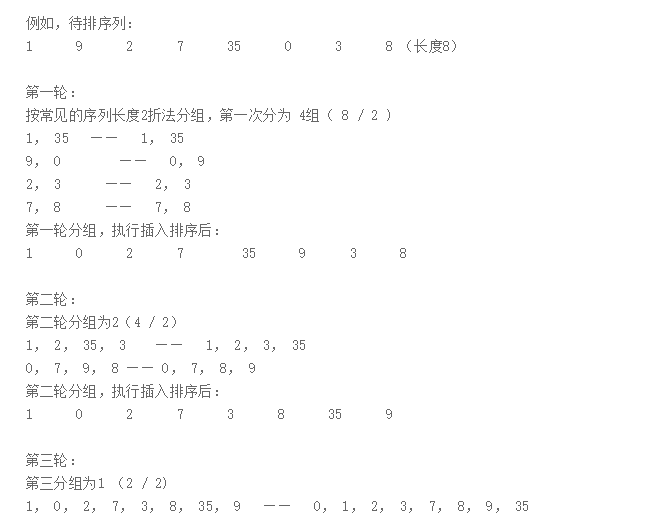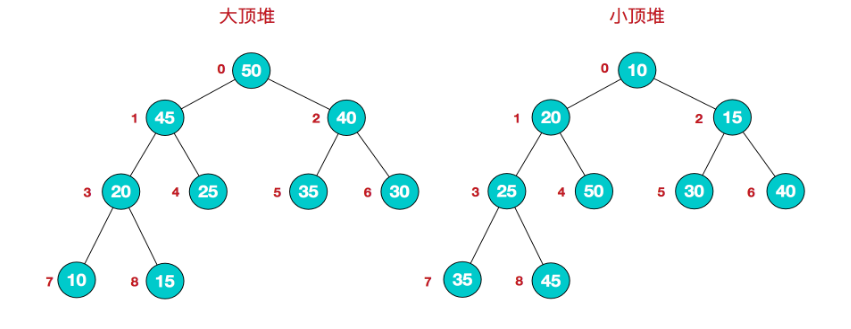# 七大经典排序（Java版）

冒泡排序：
通过相邻的两个数的比较, 根据需要决定是否将两个数互换位置, 然后将比较往前(或往后)推进. 最简单的排序算法，直接上代码。for(i=0;i<length-1;i++)
for(j=i+1;j<length;j++)
if(arrayVal[i]>arrayVal[j])
{
//置换位置
temp=arrayVal[i];
arrayVal[i]=arrayVal[j];
arrayVal[j]=temp;
}
}
View Code

"选择排序"就是第0个逐步和后面全部的比,比完0位置就得到最小的数,紧接着再从1位置对比后面的元素,以此类推,逐步得到从小到大的值.for(int i = 0; i < arr.length - 1; i++) {        int k = i;    for(int j = k + 1; j < arr.length; j++){        if( arr[j] < arr[k]){        k =j;      }        //在内层循环结束，也就是找到本轮循环的最小的数以后，再进行交换    if( i != k) {     int temp= arr[i];    arr[i] = arr[k];    arr[k] = temp;    }
View Code

首先对数组的前两个数据进行从小到大的排序。
接着将第三个数据与排好序的两个数据比较，将第三个数据插入合适的位置。
然后将第四个数据插入到已排好序的前3个数据中。
....

// 第1个数肯定是有序的，从第2个数开始遍历，依次插入有序序列

public  static void insertionSort(int[] a){

for(int i = 1 ; i < a.length; i++){

int temp = a[i];
int j = i - 1;
while( j >= 0 && temp < a[j]){
a[j+1] = a[j];
j--;
}
a[j+1] = temp;
}

Shell排序算法：（希尔排序、缩小增量排序）：

Shell排序是基于插入排序的思想。
1.将有n个元素的数组分成n/2个数字序列，第1个数据和第n/2+1个数据为一对，...
2.一次循环使每一个序列对排好顺序。
3.然后，变为n/4个序列，在次排序。
4.直到序列变为1个。//希尔排序
public static void shellSort(int a[]){

for(int r = a.length/2 ; r >= 1; r/=2 ){

for(int i = r; i < a.length ; i++){

int temp = a[i];
int j = i - r;

while(j >= 0 && temp < a[j]){
a[j+r] = a[j];
j -= r;
}

a[j+r] = temp;
}
}
}

1.在待排序的元素任取一个元素作为基准(通常选第一个元素，但最的选择方法是从待排序元素中随机选取一个作为基准)，称为基准元素；
2.将待排序的元素进行分区，比基准元素大的元素放在它的右边，比其小的放在它的左边；
3.对左右两个分区重复以上步骤直到所有元素都是有序的。

public static void quickSort3(int arr[],int _left,int _right){

int left = _left;
int right = _right;
int temp = 0;

if(left <= right){   //待排序的元素至少有两个的情况

temp = arr[left];  //待排序的第一个元素作为基准元素
while(left != right){   //从左右两边交替扫描，直到left = right
while(right > left && arr[right] >= temp){
right --;        //从右往左扫描，找到第一个比基准元素小的元素
}
arr[left] = arr[right];
while(left < right && arr[left] <= temp){
left ++;         //从左往右扫描，找到第一个比基准元素大的元素
}

arr[right] = arr[left];
}
arr[right] = temp;    //基准元素归位
quickSort3(arr,_left,left-1);  //对基准元素左边的元素进行递归排序
quickSort3(arr, right+1,_right);  //对基准元素右边的进行递归排序

}
}

堆排序是利用堆这种数据结构而设计的一种排序算法，堆排序是一种选择排序，它的最坏，最好，平均时间复杂度均为O(nlogn)，它也是不稳定排序。首先简单了解下堆结构。
堆：
堆是具有以下性质的完全二叉树：
每个结点的值都大于或等于其左右孩子结点的值，称为大顶堆；或者每个结点的值都小于或等于其左右孩子结点的值，称为小顶堆。堆排序的基本思想：将待排序序列构造成一个大顶堆，此时，整个序列的最大值就是堆顶的根节点。将其与末尾元素进行交换，此时末尾就为最大值。然后将剩余n-1个元素重新构造成一个堆，这样会得到n个元素的次小值。如此反复执行，便能得到一个有序序列了。

堆排序的基本步骤：

1.构造初始堆。将给定无序序列构造成一个大顶堆（一般升序采用大顶堆，降序采用小顶堆)。

2.将堆顶元素与末尾元素进行交换，使末尾元素最大。然后继续调整堆，再将堆顶元素与末尾元素交换，得到第二大元素。如此反复进行交换、重建、交换。

public class HeapSort {

public static void main(String []args){
int []arr = {9,8,7,6,5,4,3,2,1};
sort(arr);
System.out.println(Arrays.toString(arr));
}

public static void sort(int []arr){
//1.构建大顶堆
for(int i=arr.length/2-1;i>=0;i--){
}

for(int j=arr.length-1;j>0;j--){
swap(arr,0,j);
}
}

// 调整大顶堆
public static void adjustHeap(int []arr,int i,int length){
int temp = arr[i];
for(int k=i*2+1;k<length;k=k*2+1){
if(k+1<length && arr[k]<arr[k+1]){
k++;
}
if(arr[k] >temp){//如果子节点大于父节点，将子节点值赋给父节点
arr[i] = arr[k];
i = k;
}else{
break;
}
}
arr[i] = temp;
}
//交换元素
public static void swap(int []arr,int a ,int b){
int temp=arr[a];
arr[a] = arr[b];
arr[b] = temp;
}
}
归并排序
归并排序（MERGE-SORT）是利用归并的思想实现的排序方法，该算法采用经典的分治（divide-and-conquer）策略（分治法将问题分(divide)成一些小的问题然后递归求解，而治(conquer)的阶段则将分的阶段得到的各答案"修补"在一起，即分而治之)。

public class MergeSort {

public static void main(String []args){
int []arr = {9,8,7,6,5,4,3,2,1};
sort(arr);
System.out.println(Arrays.toString(arr));
}

public static void sort(int []arr){
int []temp = new int[arr.length];//在排序前，先建好一个长度等于原数组长度的临时数组，避免递归中频繁开辟空间
sort(arr,0,arr.length-1,temp);
}

private static void sort(int[] arr,int left,int right,int []temp){
if(left<right){
int mid = (left+right)/2;
sort(arr,left,mid,temp);//左边归并排序，使得左子序列有序
sort(arr,mid+1,right,temp);//右边归并排序，使得右子序列有序
merge(arr,left,mid,right,temp);//将两个有序子数组合并操作
}
}

private static void merge(int[] arr,int left,int mid,int right,int[] temp){
int i = left;
int j = mid+1;
int t = 0;
while (i<=mid && j<=right){
if(arr[i]<=arr[j]){
temp[t++] = arr[i++];
}else {
temp[t++] = arr[j++];
}
}
while(i<=mid){
temp[t++] = arr[i++];
}
while(j<=right){
temp[t++] = arr[j++];
}
t = 0;
//将temp中的元素全部拷贝到原数组中
while(left <= right){
arr[left++] = temp[t++];
}
}
}

 排序算法 平均速度 最坏情况 是否稳定 冒泡排序 O( n^2) O( n^2) 稳定 快速排序 O(nlogn) O( n^2) 不稳定 选择排序 O( n^2) O( n^2) 不稳定 插入排序 O( n^2) O( n^2) 稳定 堆排序 O(nlogn) O(nlogn) 不稳定 Shell排序 O( n^(3/2) ) O( n^2) 不稳定 合并排序 O(nlogn) O(nlogn) 稳定

posted @ 2018-06-08 19:18  安否  阅读(2698)  评论(3编辑  收藏